An Introduction to Quantum Computation and Quantum Communication

Rob Pike
Bell Labs
Lucent Technologies
rob@plan9.bell-labs.com June 23, 2000

Introduction

An analogy:

Newtonian physics is an approximation to Einsteinian physics (general relativity).

Classical physics is an approximation to quantum mechanics.

Classical information is an approximation to quantum information.

In each case, the approximation excludes important details but serves well for many purposes.

In each case, removing the approximation requires deeper understanding and harder math, but results in a truer picture of Nature and may enable new technologies.

Yes, Nature: we're beginning to understand that information is a physical concept.

What approximation do we remove?

Relativity: we remove (among others) the approximation that we are traveling much slower than light.

Quantum mechanics: we remove (among others) the approximation that we are manipulating things much larger than atoms.

Quantum computation: we remove (among others) the approximation that the elements of information are independently manipulable.

Why would we care?

That approximation means that we can look at one bit in a register without affecting the other bits.

Why remove that approximation? Because it limits the power of the computer. (Keep in mind the analogies.)

Also, getting ahead of ourselves, that approximation turns out to be troublesome in representing information quantum mechanically.

Why would we do that?

We're running out of particles.

The insulators in CMOS transistors can't get much smaller or the insulating layers will stop insulating (at around 6 atoms thick). (Maybe before 2010.)

In optical fiber, we use ten thousand or so photons to represent a bit. There's a Moore's law for fiber, too, and we'll soon run out of photons. (Maybe before 2010.)

Quantum mechanical effects will become important in just a few years!

Currently, we work in the classical information regime. That won't last. We'd better come to understand quantum information.

Of course, this version of the story isn't how quantum computation came to be. (Keep in mind the analogies.) So let's back up and tell a more historical story, to introduce the ideas.

Feynman's Question

In a couple of papers in the 1980s, Feynman asked and began to answer the following question:
Is it feasible for a computer to simulate a physical system perfectly?

The answer appears to be, "No". A classical computer seems to need time exponential in n to predict precisely the behavior of a general quantum mechanical system of n particles. (Yet nature manages to do it in real time.)

Briefly, a quantum mechanical system of n particles is represented by a wave function in a Hilbert space of dimension exponential in n. We really do need that much dimensionality to represent all possible behaviors of the system.

Less briefly...

The Nature of Quantum Reality

The two-slit experiment.1. Single photon still produces interference pattern!

2. Ask which slit photon passes - pattern disappears!

Interpretation

The photon can go through either slit, or both; its state embodies both possibilities.

If we ask which slit it went through, there must be an answer, and the system must decide:
Asking the question changes the state of the system from both possibilities to exactly one.

The Quantum Measurement Postulate
When you make a measurement, the system makes a random selection among the possible answers and chooses one. After the measurement, the system is in the state that always gives that answer; the possibility of other answers is gone.

Do the measurement again (sufficiently quickly) and you'll get the same answer.

Quantum mechanics in two slides (I)

The state of a QM system is described by its wave function,an oscillating complex-valued function defined over all of space.can interfere with itself.

Quantum mechanics is linear. We can create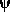by linear combination, e.g.:For well-defined states, e.g. up, we use the notationas inTheare complex coefficients that must normalize; if the |> states are orthogonal, themust satisfy:These are called probability amplitudes and(note the square) is the probability that if we make a measurement of the system, we will find it in stateQuantum mechanics in two slides (II)

The quantum measurement postulate in math:

If we make a measurement on a system with wave functionand find it's in state i, the wave function is nowMath aside: the i are the eigenvalues corresponding to eigenvectorsof the operator (e.g. energy) defining the measurement.

See for yourself

Light can be linearly polarized: its vibrations can lie in a plane, say horizontally or vertically. Represent these two possibilities asand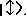We call these orthogonal states a basis of the system.

Plain light is a mixture of these polarizations and in fact a single photon can be a mixture. For example, light polarized at 45° is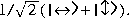Light can also be circularly polarized: circular polarization can be created from linear as follows:This is another orthogonal basis of the polarization.

We can demonstrate that light obeys the quantum measurement postulate using three linear polarizing filters and an overhead projector....

A few points about wave functions

1. Quantum mechanics is a linear theory: we can create linear superpositions of wave functions, provided we keep the probability amplitudes normalized.

2. The quantum measurement postulate can be described as the wave function `collapsing' to the basis state corresponding to the outcome of the experiment.

3. We cannot discover the full quantum state of a system, only the squared probability amplitudesTheare the projections of the system onto the basis states and are complex-valued.

4: We cannot clone an unknown quantum state. There are no quantum wires. (Proof a little later.)

Bits and Qubits

A bit is in one of two states, 0 and 1, represented by e.g. the state of a switch or a voltage.

To map this to quantum mechanics, choose two orthogonal states (e.g. horizontal and vertical polarization) and label theseandThe state maps to a Boolean 0 or 1.

A qubit is a parcel of information represented by such a system. Because quantum mechanics is linear, unlike Boolean algebra, a qubit can be not just the valueand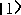but any complex linear superposition that satisfies the normalization condition.

For example, a qubit might bea horizontally polarized photon; or it might bea vertically polarized one, or it might bea right circularly polarized one, or any other linear combination with appropriate normalization.

The interpretation of Qubits

A bit represents one of two points, but a qubit represents any point on the unit circle in the complex plane.To ask the state of the qubit is to ask whether it isorand by QMP it must decide. Therefore, when we measure a qubit, we can only ever getor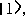corresponding to Boolean 0 or 1. But until we ask, it can be an arbitrary mixture ofandMultiple bits and qubits

N bits can representinteger values.

N qubits can represent any complex vector of unit length indimensions, one dimension for each possible classical state. A spectacularly larger set of values!

3 bits can represent any one of 000, 001, 010, ..., 111.

3 qubits can represent any value of the form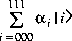as long as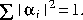For example, a 3-qubit register might have the valueThere is no classical analogue of this sort of state. The register represents two (or up to 2^N) different values simultaneously!

The register could be in the `pure' stateorbut the overwhelming majority of possible states are not pure.

Entanglement

Two classical bits can be 00 or 01 or 10 or 11. We can ask the value of the first bit without affecting the second bit.

Two qubits could be in the stateThe first qubit is neithernorIt's not even a superposition ofandbecause the state is not separable: the value of the first qubit is entangled with the value of the second.

We can't discover value of first qubit affecting the second. Say we measure it and get 0; by QMP that means the state of the system is now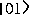and therefore the second qubit is nowBut it wasn'tbefore; it was entangled with the first qubit.

This is another very different feature of quantum information.

1. An entanglement by definition involves multiple qubits; this is not an entangled state:2. A superposition is not necessarily entangled. ConsiderWe can measure the first qubit without affecting the second.

Compare the two above with this truly entangled state:Proof of the no cloning theorem

Proof by contradiction. Assume we have a box that will take an arbitrary qubit and create a copy. Giventhe result will be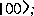giventhe result will beGiven the arbitrary statewe want as output two separable qubits like this:But quantum mechanics is linear, so applying the box to our state will produceUnless one oforis zero, this is not the desired state; it is entangled. Therefore the cloning box cannot exist.

Similarly, an unknown quantum state cannot be deleted without affecting the rest of the system.

Conservation of information.

This theorem means: no wires, no oscilloscope probes, no debugging print statements. Note: this theorem doesn't apply once we measure the qubits, since the result is a pure state.

Computation

Classically, we put n bits into a calculation and get m bits out:A quantum computer can't create or destroy qubits during the calculation, so m must equal n:The quantum computer is an operator that maps n input qubits to n output qubits. Recall that n qubits represent a unit vector pointing to the surface of a sphere in complex space ofdimensions. Therefore the QC is a kind of rotation; it can be represented by a rotation matrix in complexspace; such matrices are called unitary.

Quantum systems evolve by unitary operations, and all steps in a quantum calculation must be unitary.

The final measurement step does not need to be unitary, since we can throw data away at the end.

A quantum gate

A quantum gate is a unitary operator, so number of bits in equals number of bits out. No AND or OR, but instead e.g., a controlled-NOT, which inverts B if A is 1:It's a rotation, so reversible: given the output, we can recover the input.

Other quantum gates include controlled-controlled-NOT, square root of NOT, and other exotica.

Reversibility has the side effect that, in principle, it means a quantum gate can use zero energy (but might take arbitrarily long).

Reversibility has the undesired side effect that we are forbidden from using latches, feedback, or rewritable memory.

The big picture

A quantum computer looks like this, taking n input qubits, the register V, and producing n output qubits, the register W:The input register can be prepared as a superposition of states, e.g. an equal superposition of all integers from 0 toThe computer then calculates in parallel the function applied to all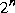integers simultaneously.

From QMP, when we measure W, it will choose a Boolean for each bit of the output register according to the resulting entangled wave function of the output qubits.

Design F so that it maximizes the probability that the output we measure is the answer we want.

The big picture continued

Measuring the output collapses the wave function: get Boolean values for all the qubits in W. The result is one of the possible outputs.

Imagine that F is (integer) square rootPrepare V as the superposition of all integers from 0 torun the computer, then measure W. Result will square root of some number between 0 andThe square root of any such number, with equal probability.

F calculates the square roots of all the integers in parallel, but QMP says we can only find out about one.

For real problems, arrange F so the probability amplitudes of the output state strongly favor the desired output from F.

Recall the double-slit experiment. Quantum computers are like huge multidimensional arrays of slits that generate interference patterns in the wave functions. Design the array right, and the pattern solves your problem.

A quantum computer is probabilistic: we may need to run it multiple times before we get the answer we want.

Shor's algorithm, simplified (I)

Peter Shor showed how to design a quantum computer to calculate the factors of an integer in polynomial time, theoretically breaking RSA.

We want to factor N, that is, find A and B such that AB = N.

Trick: find distinct x and y such that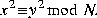Thenso one must contain a factor, which we can find by e.g. gcd(x-y, N).

Next, take y to be 1, so ifand r is even then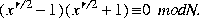Then r is the period of the functionin a.

Shor's algorithm, simplified (II)

Looking for pair x, r such thatGreatly simplifying, algorithm builds a superposition of all integers x < N, then calculatesfor all a in parallel.

Discover the periods using a (quantum) FFT on the resulting entanglement. The final state is (sort of) an entanglement of all valid x, r pairs.

Finally, measure the output register. QMP says it must choose one x, r pair, and we can factor.

With small probability the output may be zero; if so, we run it again.

Not much like a regular computer program!

What else can we do?

Shor's algorithm
factors in polynomial time.

(We expect to get a non-zero result in a small number of runs.) It's dramatically faster than any known classical algorithm;

Entanglement gives us exponential parallelism.

A few other quantum algorithms go faster than classical. Most are obscure but one is important:

Lov Grover's algorithm searches an unordered database of N elements to finds an element satisfying a given condition in square root of N time. In other words,
it searches a linear list in square root time.

Not as dramatic as the exponential speedup in Shor's algorithm, but remarkable and possibly even practical.

Decoherence

Factoring a 200-digit number using Shor requires 3500 perfectly well-behaved qubits. (Current state of the art is four entangled qubits.) But that's not the hard part.

The challenge is decoherence: the `leakage' of quantum state into the environment. Actually, it is entanglement with the environment. (Believed to be the explanation for why the macroscopic world behaves classically).

QC must be run in a sealed box without any interaction with the outside world. Otherwise the qubits will be contaminated. (This is another reason debugging could be hard.)

The required isolation is extreme; today's entangled atomic states in the lab last for about 10ns, and decoherence proceeds exponentially fast in the number of particles.

Error correction

Decoherence would be the death knell for QC, except that Shor and others discovered quantum error correction. Like classical error correction, but QEC can correct an arbitrary error in a qubit, even if we don't know its state! (Much more astonishing than repairing a bit flip in a classical message.)

Many such codes exist, e.g. 7 qubits can fully repair damage to any one qubit in the message.

QEC could compensate for decoherence and other losses if they're at a low enough rate. (Current theory ranges from .000001 to .01.) Error correcting a t-step computation involves overhead polynomial in log t.

Using Shor to factor 200 digits requires 3500 perfect qubits, 100,000 if error correction is involved.

Quantum communication

Computation may be extremely hard because it involves many qubits. But communication can work one qubit at a time. Error-correcting such states might be practical. Experiments have reliably transmitted kiloqubits per second over many kilometers of fiber, and in one case a mile of open air!

Is this a solution to the running out of photons problem? An open question. Several ways to communicate:
C: Send classical bits.
Q: Send qubits.Send qubits but also use two-way classical communication to assist.Send qubits but first prepare them by prior entanglement between sender and receiver.

Channel capacities:Entanglement is again the source of power.

EPR pairs

Einstein, Podolsky and Rosen proposed a thought experiment to show that quantum mechanics was crazy. Today we can do the EPR experiment and Einstein would have hated the result: QM is crazy.

Based on EPR, we can do stuff like teleportation, unbreakable key exchange, and high-efficiency communication.

Electron-positron annihilation produces two photons:The two must have entangled states: the polarization of one must correlate with the polarization of the other.

What if Alice measures using plane polarization? Then if Bob measures using plane polarization, he must get same answer. Ditto for circular. But.... what if Alice doesn't tell until after Bob measures?

A classical channel can be used to report how the measurement was done, and Alice and Bob can compare notes.

Using EPR pairs

Quantum key exchange: Exchange a bunch of EPR pairs. Alice reports the basis she used for her measurements; Bob checks against his, and the photons measured in the same basis get the same answer.

Cannot be tapped because tapping will destroy the entanglement. Alice can add extra `check' bits; Bob can check them to see if key has been tampered with.

After sharing an EPR pair, two classical bits can send an arbitrary quantum state from Alice to Bob. Alice combines her half of the pair with the state (say an atom), does a measurement, and sends the result to Bob. Bob uses the bits to entangle his half of the pair and the destination atom. Result is to transfer the unknown state to the atom: Teleportation!In a similar experiment, after prior sharing an EPR pair, Alice can send Bob two classical bits in one qubit. This is called superdense coding. Time is important: Alice and Bob can share and separate months before Alice decides which bits to send.EPR pairs are a new kind of data communication. There's nothing like them in classical information theory.

Quantum computation can reduce the time complexity of some calculations.

Quantum communication can reduce the communication complexity of some calculations.

Physical Realizations

Far from an exhaustive list.

Photons: 23 km. of fiber under lake Geneva, reflecting with polarization change at the end back to sender. (Gisin, U. Geneva).

Electrons: Floating on liquid helium with electrodes underneath; too early to tell (Platzman & Dykman, Bell Labs).

Atoms: Ion traps, controlling quantum state by external laser pulses (Cirac & Zoller, Austria; Kimble, Caltech). Passing qubit from atom to photon is work in progress.

Molecules: NMR on an ensemble of molecules (e.g. chloroform, trichlorethylene) (Gershenfeld, MIT).

All these have limitations. Current status: a few qubits.

Solid state: In the future. Quantum dots, ultrasmall Josephson junctions, semiconductor microcavities, ...

Conclusions

As computational elements get smaller and smaller, quantum mechanical effects will become important. Somewhat to our surprise, this may turn out to be a good thing.

Information is a physical variable, and we can use the properties of its physical manifestation to our advantage. Quantum mechanical information has deeper structure and greater power than classical information.

Studying information as a physical notion helps us understand. For example, to understand what can and cannot travel faster than light, say this: information cannot travel faster than light.

A final thought: Sixty years ago classical computers seemed as remote as quantum computers do today.

Summary

Table adapted from Bennett & DiVincenzo:Quantum speedups:

Factoring: exponential; Search: quadratic; Iteration, parity: no speed-up; Simulation of quantum systems: up to exponential.

References

Richard. P. Feynman, "Simulating Physics with Computers", International Journal of Theoretical Physics, 1982, Vol. 21, No. 6/7, pp. 467-488.

R. P. Feynman, "Quantum Mechanical Computers", Foundations of Physics, 1986, Vol. 16, No. 6, pp. 507-531.

C. H. Bennett and G. Brassard and A. K. Ekert, "Quantum Cryptography", Scientific American, October 1992, pp. 50-57.

P. W. Shor, "Quantum Computing", Documenta Mathematica, Extra Volume ICM 1998 I, pp. 467-480.

Three good overviews:

Charles H. Bennett & David P. DiVincenzo, ``Quantum information and computation'', Nature, Vol. 404, 16 Mar 2000, pp. 247-255.

A. Steane, "Quantum Computing", Reports on Progress in Physics, 1998, Vol. 61, pp. 117-173, https://xxx.lanl.gov/abs/quant-ph/9708022.

E. G. Rieffel and W. Polak, "An Introduction to Quantum Computing for Non-Physicists", 1998, https://xxx.lanl.gov/abs/quant-ph/9809016.